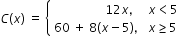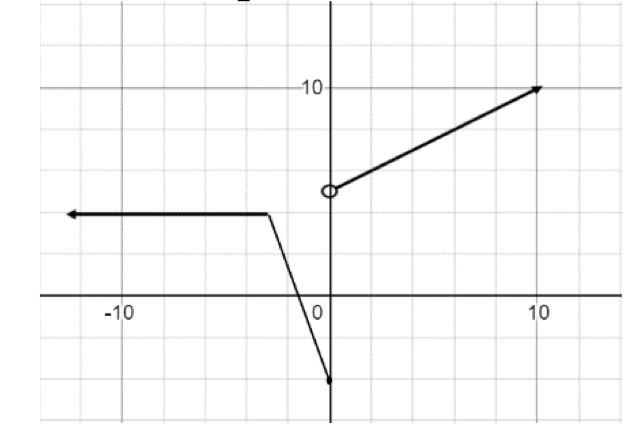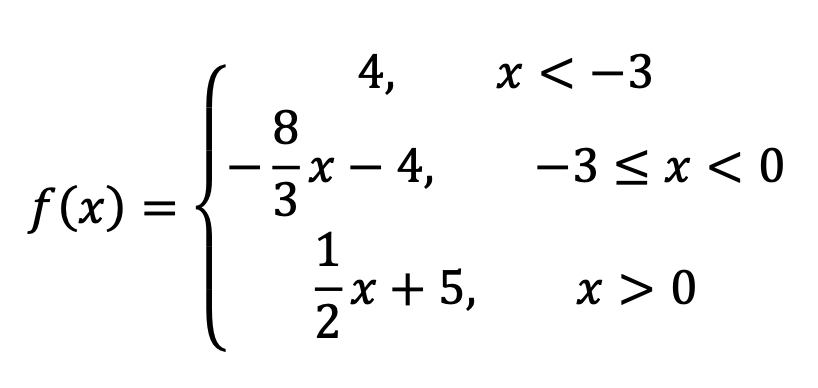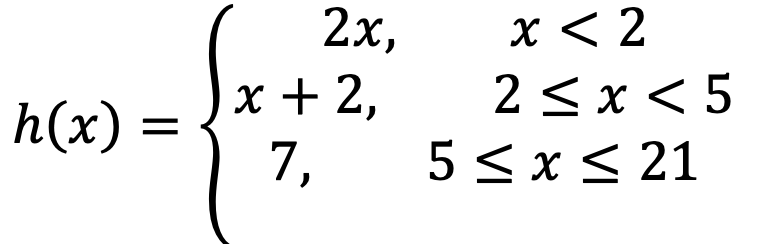# MA.912.AR.9.10Export Print
Solve and graph mathematical and real-world problems that are modeled with piecewise functions. Interpret key features and determine constraints in terms of the context.

### Examples

A mechanic wants to place an ad in his local newspaper. The cost, in dollars, of an ad x inches long is given by the following piecewise function. Find the cost of an ad that would be 16 inches long.### Clarifications

Clarification 1: Key features are limited to domain, range, intercepts, asymptotes and end behavior.

Clarification 2: Instruction includes representing the domain, range and constraints with inequality notation, interval notation or set-builder notation.

General Information
Subject Area: Mathematics (B.E.S.T.)
Strand: Algebraic Reasoning
Status: State Board Approved

## Benchmark Instructional Guide

### Terms from the K-12 Glossary

• Coordinate plane
• Domain
• Function
• Function notation
• Piecewise function
• Range (of a relation or a function)
• Rate of change
• Set-builder notation
• $x$-intercept
• $y$-intercept

### Vertical Alignment

Previous Benchmarks

Next Benchmarks

### Purpose and Instructional Strategies

In Algebra I, students worked with linear, quadratic, absolute value and exponential functions. In Math for Data and Financial Literacy, students work with piecewise functions, interpret the key features of their graphs, and determine constraints in terms of the context.
• Instruction includes real-world problems that can be modeled with piecewise functions (MTR.7.1).
• It is important for students to understand that a piecewise function is a function defined by multiple sub functions, each of which applies to a certain interval defined by the function’s domain.
• For mastery of this benchmark within this course, sub functions are limited to linear, quadratic and exponential.• Instruction includes the use of $x$-$y$ notation and function notation.
• Instruction includes representing domain, range and any constraints using words, inequality notation, set-builder notation and interval notation.
• Words
If the domain is all real numbers, it can be written as “all real numbers” or “any value of $x$, such that $x$ is a real number.”
• Inequality notation
If the domain is all values of $x$ greater than 2, it can be represented as $x$ > 2.
• Set-builder notation
If the range is all values of $y$ less than or equal to zero, it can be represented as {$y$|$y$ ≤ 0} and is read as “all values of $y$ such that $y$ is less than or equal to zero.”
• Interval notation
If the domain is all values of $x$ less than or equal to 3, it can be represented as (−∞, 3]. If the domain is all values of $x$ greater than 3, it can be represented as (3, ∞). If the range is all values greater than or equal to −1 but less than 5, it can be represented as [−1, 5).
• This benchmark expects students to interpret key features and determine constraints in terms of the context of a real-world problem (MTR.7.1).
• For the example used in the benchmark, the piecewise function models the cost, in dollars, of an ad $x$ inches long. The key features and constraints are as follows.
• The domain of a function is the complete set of possible values of the input of a function or relation. The input values in this case are the size in inches of the ad length. The minimum ad length someone could possibly have is 0 inches. The maximum ad length (based on the length of traditional newspapers in the United States) would be the length of a full page, which is approximately 21 inches. The set of inputs could be considered continuous within the minimum and maximum since the length is not limited to only whole numbers. Therefore, a reasonable domain for this context would be any length from 0 to 21 inches.
• The range of a function is the complete set of possible values of the output of a function or relation. In this case, the output is the cost of the ad. The minimum cost of an ad would be 0 if the ad size length is 0. This function uses the subset function 12$x$ when $x$ < 5. The upper bound of the cost for this subset would be 12(5), or 60, but does not include 60. Once the size of the ad reaches 5 inches in length, the subset function 60 + 8($x$ − 5) is used. The lowest cost for this subset would be 60 + 8(5 − 5) = 60 and the highest cost for that subset would be 60 + 8(21 − 5) = 188. Overall, the lowest cost would be 0 and the highest cost would be 188, so the range is all values between 0 and 188, inclusive.
• The $x$-intercept occurs when the output value is 0. The $y$-intercept occurs when the input value is 0. In this case, the $x$-intercept occurs when the cost of the ad is equal to 0. The cost of the ad is 0 when the length of the ad is 0 inches. The $C$($x$)-intercept is the starting cost of the ad when the ad length is 0. The starting cost of the ad when the ad length is 0 is 0 dollars. Therefore, the $x$-intercept and the $C$($x$)-intercept is the origin (0,0).

### Common Misconceptions or Errors

• When evaluating a piecewise function for a specific input, students may attempt to substitute the input value into the wrong subset function or may try to substitute the input value into all the subset functions.
• When graphing piecewise functions, students may forget to put an open circle at the endpoint of a subset function when the endpoint is not included in the domain of the subset function.
• Students may have trouble interpreting the range when the range is not continuous and joins two or more intervals.

• The function below models the height, $h$($x$), in inches, of water in an aquarium $x$ minutes after beginning to fill the aquarium.• Part A. Graph the function. Be sure to label your graph.
• Part B. Write a story to describe what is happening in your graph.
• Part C. Interpret the domain, range and intercepts of the function in the context of the problem.
• Part D. What will the height of the water be 2 minutes after beginning to fill the aquarium?

### Instructional Items

Instructional Item 1
• Miami airport offers several different parking options for visitors. A new short term (no more than 24 hours) parking garage charges \$1 per hour (or any part thereof) for the first two hours and after that, \$3 per hour (or any part thereof) not to exceed \$20 per day.
• Part A. Graph the function described above.
• Part B. Interpret the domain and range of the function in the context of the problem.
*The strategies, tasks and items included in the B1G-M are examples and should not be considered comprehensive.

## Related Courses

This benchmark is part of these courses.
1200340: Algebra 2 Honors (Specifically in versions: 2014 - 2015, 2015 - 2022, 2022 and beyond (current))
1200400: Foundational Skills in Mathematics 9-12 (Specifically in versions: 2014 - 2015, 2015 - 2022, 2022 and beyond (current))
1202340: Precalculus Honors (Specifically in versions: 2014 - 2015, 2015 - 2022, 2022 and beyond (current))
1200388: Mathematics for Data and Financial Literacy Honors (Specifically in versions: 2022 and beyond (current))
1200384: Mathematics for Data and Financial Literacy (Specifically in versions: 2022 and beyond (current))
1200710: Mathematics for College Algebra (Specifically in versions: 2022 and beyond (current))
1209315: Mathematics for ACT and SAT (Specifically in versions: 2022 and beyond (current))

## Related Access Points

Alternate version of this benchmark for students with significant cognitive disabilities.

## Related Resources

Vetted resources educators can use to teach the concepts and skills in this benchmark.

## Formative Assessments

Graphing a Step Function:

Students are asked to graph a step function, state the domain of the function, and name any intercepts.

Type: Formative Assessment

Airport Parking:

Students are given a graph and a verbal description of a function and are asked to describe its domain.

Type: Formative Assessment

## MFAS Formative Assessments

Airport Parking:

Students are given a graph and a verbal description of a function and are asked to describe its domain.

Graphing a Step Function:

Students are asked to graph a step function, state the domain of the function, and name any intercepts.

## Student Resources

Vetted resources students can use to learn the concepts and skills in this benchmark.

## Parent Resources

Vetted resources caregivers can use to help students learn the concepts and skills in this benchmark.